### 2.4.1.3 Band Structure Periodicity

For two wave vectors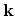and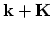the solutions of Schrödinger equation (2.68) are related to each other as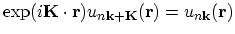. This leads to equal eigenvalues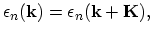(2.70)

and equal wave functions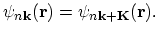(2.71)

It can be seen that each energy branch has the same period as the reciprocal lattice. As the functions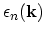are periodic, they have maxima and minima which determine the width of the bands.

It should be noted that the wave vectorin (2.66) can always be chosen in a way to belong to the first Brillouin zone because any vector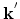out of the first Brillouin zone can be represented as the sum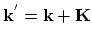, where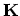is a vector of the reciprocal lattice. Using the equivalent form of Bloch's theorem: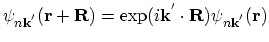(2.72)

together with (2.71) and the equality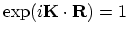one obtains (2.72) for vector. S. Smirnov: